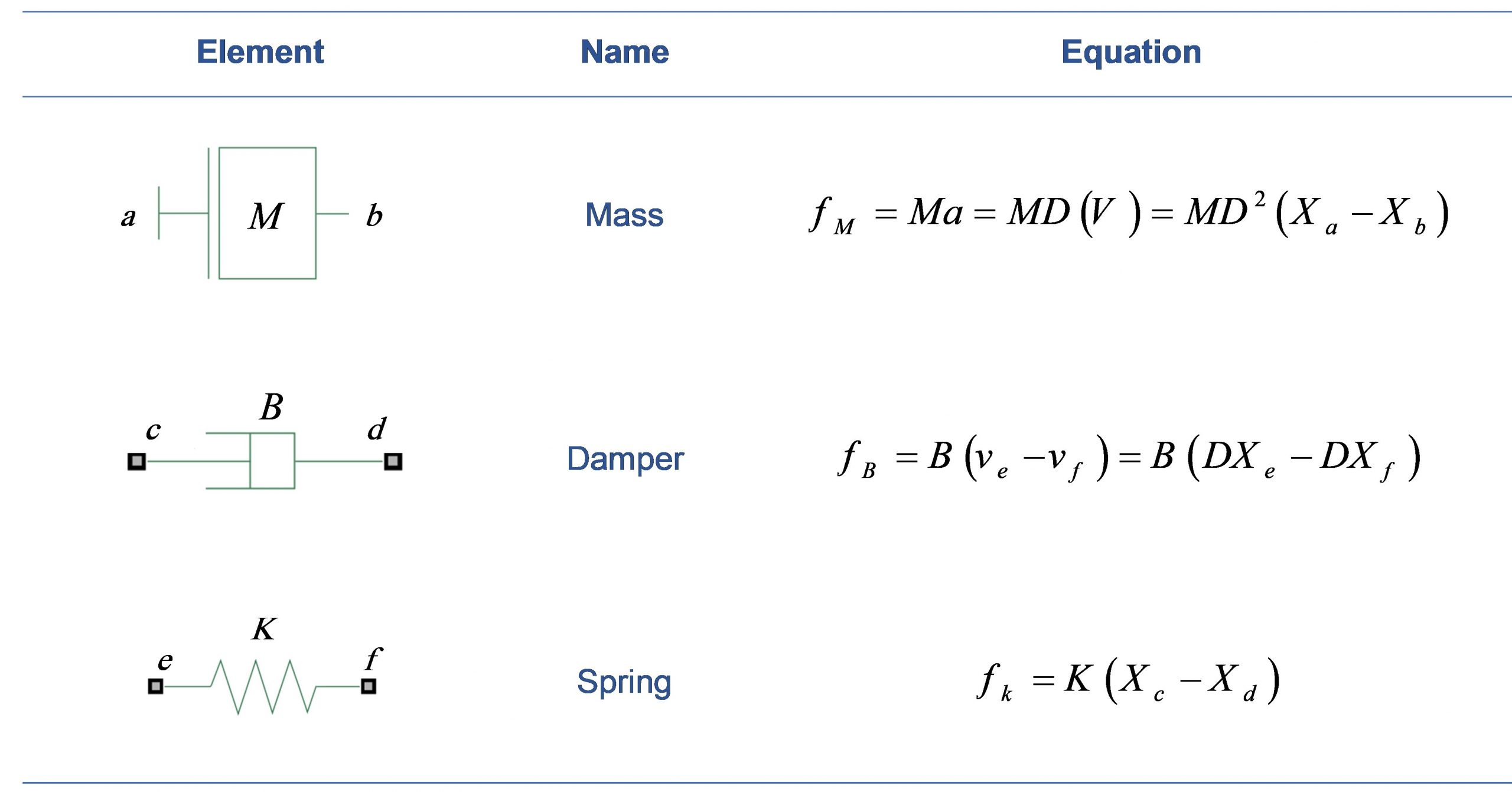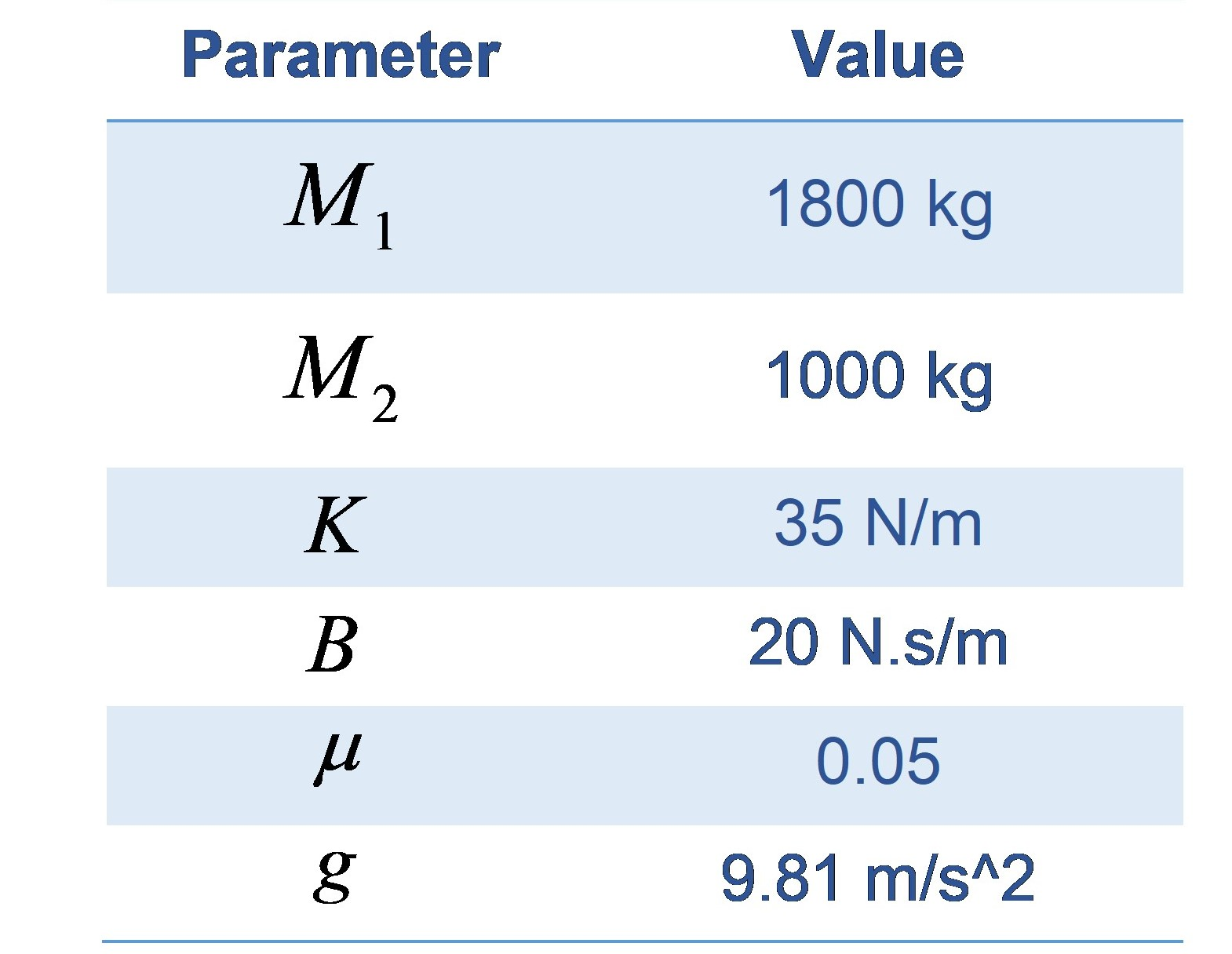# 9 Train system

A train system including the locomotive and the car can be considered as a translational mechanical system as shown in Figure 5.1.

In this model, The mass of the engine and the car are represented by M1  and M2 , respectively. The two masses are held together by a spring with stiffness K  and a damper whose coefficient is B . The force applied by the engine is represented by F and the effect of rolling friction is shown by R1 and R2. The essential elements for a translational mechanical system is shown in Table 5.1.

Considering D as the derivative function (d/dt) , and can be written as:

R1=μ g M1 DX1

R2=μ g M2 DX2

where the values of the parameters are given in Table 5.2.

Table 5.1– Essential elements for a translational mechanical systemTable 2– Value of parametersObjectives

The following objectives will be covered as you go through the experiment procedure.

• Determine the characteristic polynomial, roots and modes of the system
• Determine the system response to initial condition, impulse and other inputs
• Explain the internal and external stability of the system

Procedure

1. Derive the differential equation of the system from engine power (f(t)) to location x2 . What is the input and output of the system?

2. Determine the output when specific inputs defined in Part b to d below are fed to the system. Answer to the following questions theoretically and then verify your answers in MATLAB.

a) Derive the characteristic polynomial, roots, and modes of the system according to Step 1. How is our cabin trajectory if the engine shuts down when the train’s speed is 1m/s (Zero-input response of the system)? After how much time the train stops? What about if its speed was 10 m/s when the engine stops working?

b) If another train comes forward and suddenly hits the train, how our cabin does the cabin move (Unit Impulse Response of the system)?

c) If the engine driver releases the power clutch i.e., F(t)=Feng (1-e-0.1t)u(t) and Feng=100, after how much time does it pass before the train can travel 30km? (Zero-State Response of system, and use convolution table).

d) In the previous scenario if after 30km the speed of the train is 1m/s, how much time would be saved to travel 30km? (Total response of the system).

3. Find an initial condition for the system to eliminate the slowest none-zero characteristic mode (smallest in real part). Will the train go faster now?

4. What will happen to the cabin, if the spring breaks?

5. Discuss the physical meanings of Convolution Integral properties in this system.

6. Is the cabin BIBO-stable? If not, how the engine driver can make it stable (a bounded input that leads to an unbounded output)? In that case, what is the train’s trajectory?

7. Is this system internally stable? Why?

8. Systems with one or two roots on origin are very popular in the industry and most of the input commands are steps. How they can fix this paradox?

9. (Optional) What’s the meaning of observability and controllability? How are they related to stability?